# eigs

Subset of eigenvalues and eigenvectors

## Syntax

``d = eigs(A)``
``d = eigs(A,k)``
``d = eigs(A,k,sigma)``
``d = eigs(A,k,sigma,Name,Value)``
``d = eigs(A,k,sigma,opts)``
``d = eigs(A,B,___)``
``d = eigs(Afun,n,___)``
``````[V,D] = eigs(___)``````
``````[V,D,flag] = eigs(___)``````

## Description

example

````d = eigs(A)` returns a vector of the six largest magnitude eigenvalues of matrix `A`. This is most useful when computing all of the eigenvalues with `eig` is computationally expensive, such as with large sparse matrices.```

example

````d = eigs(A,k)` returns the `k` largest magnitude eigenvalues.```

example

````d = eigs(A,k,sigma)` returns `k` eigenvalues based on the value of `sigma`. For example, `eigs(A,k,'smallestabs')` returns the `k` smallest magnitude eigenvalues.```
````d = eigs(A,k,sigma,Name,Value)` specifies additional options with one or more name-value pair arguments. For example, `eigs(A,k,sigma,'Tolerance',1e-3)` adjusts the convergence tolerance for the algorithm.```

example

````d = eigs(A,k,sigma,opts)` specifies options using a structure.```

example

````d = eigs(A,B,___)` solves the generalized eigenvalue problem `A*V = B*V*D`. You can optionally specify `k`, `sigma`, `opts`, or name-value pairs as additional input arguments.```
````d = eigs(Afun,n,___)` specifies a function handle `Afun` instead of a matrix. The second input `n` gives the size of matrix `A` used in `Afun`. You can optionally specify `B`, `k`, `sigma`, `opts`, or name-value pairs as additional input arguments.```

example

``````[V,D] = eigs(___)``` returns diagonal matrix `D` containing the eigenvalues on the main diagonal, and matrix `V` whose columns are the corresponding eigenvectors. You can use any of the input argument combinations in previous syntaxes.```

example

``````[V,D,flag] = eigs(___)``` also returns a convergence flag. If `flag` is `0`, then all the eigenvalues converged.```

## Examples

collapse all

The matrix `A = delsq(numgrid('C',15))` is a symmetric positive definite matrix with eigenvalues reasonably well-distributed in the interval (0 8). Compute the six largest magnitude eigenvalues.

```A = delsq(numgrid('C',15)); d = eigs(A)```
```d = 6×1 7.8666 7.7324 7.6531 7.5213 7.4480 7.3517 ```

Specify a second input to compute a specific number of the largest eigenvalues.

`d = eigs(A,3)`
```d = 3×1 7.8666 7.7324 7.6531 ```

The matrix `A = delsq(numgrid('C',15))` is a symmetric positive definite matrix with eigenvalues reasonably well-distributed in the interval (0 8). Compute the five smallest eigenvalues.

```A = delsq(numgrid('C',15)); d = eigs(A,5,'smallestabs')```
```d = 5×1 0.1334 0.2676 0.3469 0.4787 0.5520 ```

Create a 1500-by-1500 random sparse matrix with a 25% approximate density of nonzero elements.

```n = 1500; A = sprand(n,n,0.25);```

Find the LU factorization of the matrix, returning a permutation vector `p` that satisfies `A(p,:) = L*U`.

`[L,U,p] = lu(A,'vector');`

Create a function handle `Afun` that accepts a vector input `x` and uses the results of the LU decomposition to, in effect, return `A\x`.

`Afun = @(x) U\(L\(x(p)));`

Calculate the six smallest magnitude eigenvalues using `eigs` with the function handle `Afun`. The second input is the size of `A`.

`d = eigs(Afun,1500,6,'smallestabs')`
```d = 6×1 complex 0.1423 + 0.0000i 0.4859 + 0.0000i -0.3323 - 0.3881i -0.3323 + 0.3881i 0.1019 - 0.5381i 0.1019 + 0.5381i ```

`west0479` is a real-valued 479-by-479 sparse matrix with both real and complex pairs of conjugate eigenvalues.

Load the `west0479` matrix, then compute and plot all of the eigenvalues using `eig`. Since the eigenvalues are complex, `plot` automatically uses the real parts as the x-coordinates and the imaginary parts as the y-coordinates.

```load west0479 A = west0479; d = eig(full(A)); plot(d,'+')```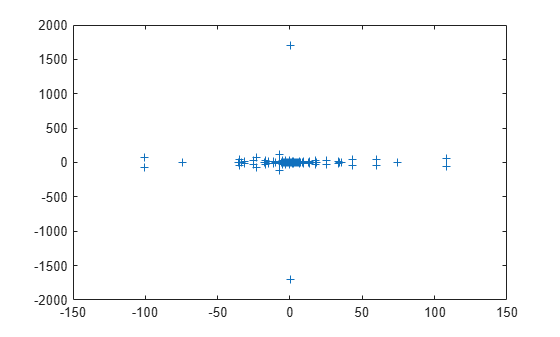The eigenvalues are clustered along the real line (x-axis), particularly near the origin.

`eigs` has several options for `sigma` that can pick out the largest or smallest eigenvalues of varying types. Compute and plot some eigenvalues for each of the available options for `sigma`.

```figure plot(d, '+') hold on la = eigs(A,6,'largestabs'); plot(la,'ro') sa = eigs(A,6,'smallestabs'); plot(sa,'go') hold off legend('All eigenvalues','Largest magnitude','Smallest magnitude') xlabel('Real axis') ylabel('Imaginary axis')```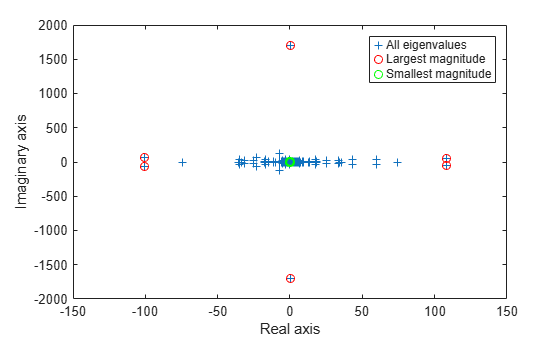```figure plot(d, '+') hold on ber = eigs(A,4,'bothendsreal'); plot(ber,'r^') bei = eigs(A,4,'bothendsimag'); plot(bei,'g^') hold off legend('All eigenvalues','Both ends real','Both ends imaginary') xlabel('Real axis') ylabel('Imaginary axis')```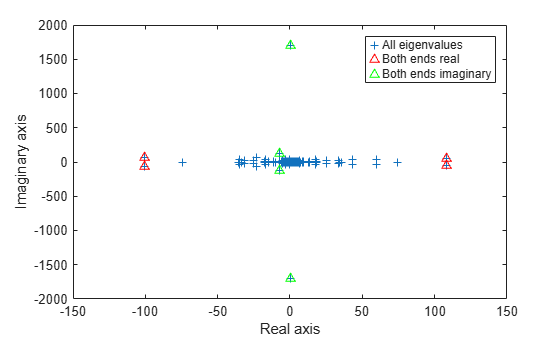```figure plot(d, '+') hold on lr = eigs(A,3,'largestreal'); plot(lr,'ro') sr = eigs(A,3,'smallestreal'); plot(sr,'go') li = eigs(A,3,'largestimag','SubspaceDimension',45); plot(li,'m^') si = eigs(A,3,'smallestimag','SubspaceDimension',45); plot(si,'c^') hold off legend('All eigenvalues','Largest real','Smallest real','Largest imaginary','Smallest imaginary') xlabel('Real axis') ylabel('Imaginary axis')```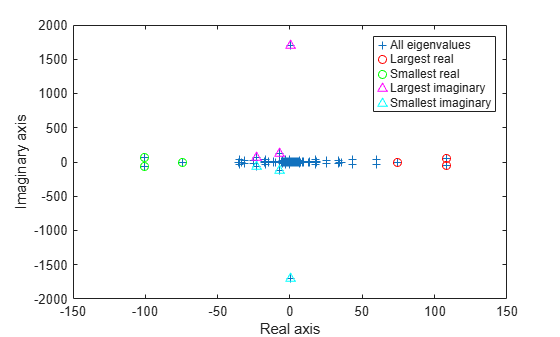Create a symmetric positive definite sparse matrix.

`A = delsq(numgrid('C', 150));`

Compute the six smallest real eigenvalues using `'smallestreal'`, which employs a Krylov method using `A`.

```tic d = eigs(A, 6, 'smallestreal')```
```d = 6×1 0.0013 0.0025 0.0033 0.0045 0.0052 0.0063 ```
`toc`
```Elapsed time is 2.703097 seconds. ```

Compute the same eigenvalues using `'smallestabs'`, which employs a Krylov method using the inverse of `A`.

```tic dsm = eigs(A, 6, 'smallestabs')```
```dsm = 6×1 0.0013 0.0025 0.0033 0.0045 0.0052 0.0063 ```
`toc`
```Elapsed time is 0.317597 seconds. ```

The eigenvalues are clustered near zero. The `'smallestreal'` computation struggles to converge using `A` since the gap between the eigenvalues is so small. Conversely, the `'smallestabs'` option uses the inverse of `A`, and therefore the inverse of the eigenvalues of `A`, which have a much larger gap and are therefore easier to compute. This improved performance comes at the cost of factorizing `A`, which is not necessary with `'smallestreal'`.

Compute eigenvalues near a numeric `sigma` value that is nearly equal to an eigenvalue.

The matrix `A = delsq(numgrid('C',30))` is a symmetric positive definite matrix of size 632 with eigenvalues reasonably well-distributed in the interval (0 8), but with 18 eigenvalues repeated at 4.0. To calculate some eigenvalues near 4.0, it is reasonable to try the function call `eigs(A,20,4.0)`. However, this call computes the largest eigenvalues of the inverse of `A - 4.0*I`, where `I` is an identity matrix. Because 4.0 is an eigenvalue of `A`, this matrix is singular and therefore does not have an inverse. `eigs` fails and produces an error message. The numeric value of `sigma` cannot be exactly equal to an eigenvalue. Instead, you must use a value of `sigma` that is near but not equal to 4.0 to find those eigenvalues.

Compute all of the eigenvalues using `eig`, and the 20 eigenvalues closest to 4 - 1e-6 using `eigs` to compare results. Plot the eigenvalues calculated with each method.

```A = delsq(numgrid('C',30)); sigma = 4 - 1e-6; d = eig(A); D = sort(eigs(A,20,sigma));```
```plot(d(307:326),'ks') hold on plot(D,'k+') hold off legend('eig(A)','eigs(A,20,sigma)') title('18 Repeated Eigenvalues of A')```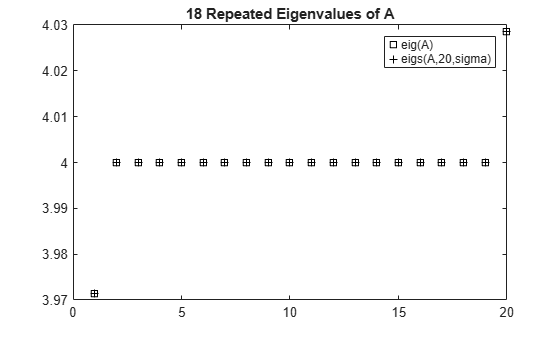Create sparse random matrices `A` and `B` that both have low densities of nonzero elements.

```B = sprandn(1e3,1e3,0.001) + speye(1e3); B = B'*B; A = sprandn(1e3,1e3,0.005); A = A+A';```

Find the Cholesky decomposition of matrix `B`, using three outputs to return the permutation vector `s` and test value `p`.

```[R,p,s] = chol(B,'vector'); p```
```p = 0 ```

Since `p` is zero, `B` is a symmetric positive definite matrix that satisfies `B(s,s) = R'*R`.

Calculate the six largest magnitude eigenvalues and eigenvectors of the generalized eigenvalue problem involving `A` and `R`. Since `R` is the Cholesky factor of `B`, specify `'IsCholesky'` as `true`. Furthermore, since `B(s,s) = R'*R` and thus `R = chol(B(s,s))`, use the permutation vector `s` as the value of `'CholeskyPermutation'`.

```[V,D,flag] = eigs(A,R,6,'largestabs','IsCholesky',true,'CholeskyPermutation',s); flag```
```flag = 0 ```

Since `flag` is zero, all of the eigenvalues converged.

## Input Arguments

collapse all

Input matrix, specified as a square matrix. `A` is typically, but not always, a large and sparse matrix.

If `A` is symmetric, then `eigs` uses a specialized algorithm for that case. If `A` is nearly symmetric, then consider using ```A = (A+A')/2``` to make `A` symmetric before calling `eigs`. This ensures that `eigs` calculates real eigenvalues instead of complex ones.

Data Types: `double`
Complex Number Support: Yes

Input matrix, specified as a square matrix of the same size as `A`. When `B` is specified, `eigs` solves the generalized eigenvalue problem `A*V = B*V*D`.

If `B` is symmetric positive definite, then `eigs` uses a specialized algorithm for that case. If `B` is nearly symmetric positive definite, then consider using `B = (B+B')/2` to make `B` symmetric before calling `eigs`.

When `A` is scalar, you can specify `B` as an empty matrix `eigs(A,[],k)` to solve the standard eigenvalue problem and disambiguate between `B` and `k`.

Data Types: `double`
Complex Number Support: Yes

Number of eigenvalues to compute, specified as a positive scalar integer.

Example: `eigs(A,2)` returns the two largest eigenvalues of `A`.

Type of eigenvalues, specified as one of the values in the table.

sigma

Description

sigma (R2017a and earlier)

scalar (real or complex, including 0)

The eigenvalues closest to the number `sigma`.

No change

`'largestabs'` (default)

Largest magnitude.

`'lm'`

`'smallestabs'`

Smallest magnitude. Same as ```sigma = 0```.

`'sm'`

`'largestreal'`

Largest real.

`'lr'`, `'la'`

`'smallestreal'`

Smallest real.

`'sr'`, `'sa'`

`'bothendsreal'`

Both ends, with `k/2` values with largest and smallest real part respectively (one more from high end if `k` is odd).

`'be'`

For nonsymmetric problems, `sigma` also can be:

sigma

Description

sigma (R2017a and earlier)

`'largestimag'`

Largest imaginary part.

`'li'` if `A` is complex.

`'smallestimag'`

Smallest imaginary part.

`'si'` if `A` is complex.

`'bothendsimag'`

Both ends, with `k/2` values with largest and smallest imaginary part (one more from high end if `k` is odd).

`'li'` if `A` is real.

Example: `eigs(A,k,1)` returns the `k` eigenvalues closest to 1.

Example: `eigs(A,k,'smallestabs')` returns the `k` smallest magnitude eigenvalues.

Data Types: `double` | `char` | `string`

Options structure, specified as a structure containing one or more of the fields in this table.

### Note

Use of the options structure to specify options is not recommended. Use name-value pairs instead.

Option FieldDescriptionName-Value Pair
`issym`

Symmetry of `Afun` matrix.

`'IsFunctionSymmetric'`
`tol`

Convergence tolerance.

`'Tolerance'`
`maxit`

Maximum number of iterations.

`'MaxIterations'`
`p`

Number of Lanczos basis vectors.

`'SubspaceDimension'`
`v0`

Starting vector.

`'StartVector'`
`disp`

Diagnostic information display level.

`'Display'`
`fail`Treatment of nonconverged eigenvalues in the output.`'FailureTreatment'`
`spdB`Is `B` symmetric positive definite?`'IsSymmetricDefinite'`
`cholB`

Is `B` the Cholesky factor `chol(B)`?

`'IsCholesky'`
`permB`

Specify the permutation vector `permB` if sparse `B` is really `chol(B(permB,permB))`.

`'CholeskyPermutation'`

Example: `opts.issym = 1, opts.tol = 1e-10` creates a structure with values set for the fields `issym` and `tol`.

Data Types: `struct`

Matrix function, specified as a function handle. The function `y = Afun(x)` must return the proper value depending on the `sigma` input:

• `A*x` — If `sigma` is unspecified or any text option other than `'smallestabs'`.

• `A\x` — If `sigma` is `0` or `'smallestabs'`.

• `(A-sigma*I)\x` — If `sigma` is a nonzero scalar (for standard eigenvalue problem).

• `(A-sigma*B)\x` — If `sigma` is a nonzero scalar (for generalized eigenvalue problem).

For example, the following `Afun` works when calling `eigs` with `sigma = 'smallestabs'`:

```[L,U,p] = lu(A,'vector'); Afun = @(x) U\(L\(x(p))); d = eigs(Afun,100,6,'smallestabs')```

For a generalized eigenvalue problem, add matrix `B` as follows (`B` cannot be represented by a function handle):

`d = eigs(Afun,100,B,6,'smallestabs')`

`A` is assumed to be nonsymmetric unless `'IsFunctionSymmetric'` (or `opts.issym`) specifies otherwise. Setting `'IsFunctionSymmetric'` to `true` ensures that `eigs` calculates real eigenvalues instead of complex ones.

For information on how to provide additional parameters to the `Afun` function, see Parameterizing Functions.

### Tip

Call `eigs` with the `'Display'` option turned on to see what output is expected from `Afun`.

Size of square matrix `A` that is represented by `Afun`, specified as a positive scalar integer.

### Name-Value Pair Arguments

Specify optional comma-separated pairs of `Name,Value` arguments. `Name` is the argument name and `Value` is the corresponding value. `Name` must appear inside quotes. You can specify several name and value pair arguments in any order as `Name1,Value1,...,NameN,ValueN`.

Example: ```d = eigs(A,k,sigma,'Tolerance',1e-10,'MaxIterations',100)``` loosens the convergence tolerance and uses fewer iterations.

#### General Options

collapse all

Convergence tolerance, specified as the comma-separated pair consisting of `'Tolerance'` and a positive real numeric scalar.

Example: ```s = eigs(A,k,sigma,'Tolerance',1e-3)```

Maximum number of algorithm iterations, specified as the comma-separated pair consisting of `'MaxIterations'` and a positive integer.

Example: ```d = eigs(A,k,sigma,'MaxIterations',350)```

Maximum size of Krylov subspace, specified as the comma-separated pair consisting of `'SubspaceDimension'` and a nonnegative integer. The `'SubspaceDimension'` value must be greater than or equal to `k + 1` for real symmetric problems, and `k + 2` otherwise, where `k` is the number of eigenvalues.

The recommended value is `p >= 2*k`, or for real nonsymmetric problems, `p >= 2*k+1`. If you do not specify a `'SubspaceDimension'` value, then the default algorithm uses at least `20` Lanczos vectors.

For problems where `eigs` fails to converge, increasing the value of `'SubspaceDimension'` can improve the convergence behavior. However, increasing the value too much can cause memory issues.

Example: ```d = eigs(A,k,sigma,'SubspaceDimension',25)```

Initial starting vector, specified as the comma-separated pair consisting of `'StartVector'` and a numeric vector.

The primary reason to specify a different random starting vector is when you want to control the random number stream used to generate the vector.

### Note

`eigs` selects the starting vectors in a reproducible manner using a private random number stream. Changing the random number seed does not affect the starting vector.

Example: ```d = eigs(A,k,sigma,'StartVector',randn(m,1))``` uses a random starting vector that draws values from the global random number stream.

Data Types: `double`

Treatment of nonconverged eigenvalues, specified as the comma-separated pair consisting of `'FailureTreatment'` and one of the options: `'replacenan'`, `'keep'`, or `'drop'`.

The value of `'FailureTreatment'` determines how `eigs` displays nonconverged eigenvalues in the output.

Option

Affect on output

`'replacenan'`

Replace nonconverged eigenvalues with `NaN` values.

`'keep'`

Include nonconverged eigenvalues in the output.

`'drop'`

Remove nonconverged eigenvalues from the output. This option can result in `eigs` returning fewer eigenvalues than requested.

Example: ```d = eigs(A,k,sigma,'FailureTreatment','drop')``` removes nonconverged eigenvalues from the output.

Data Types: `char` | `string`

Toggle for diagnostic information display, specified as the comma-separated pair consisting of `'Display'` and a numeric or logical `1` (`true`) or `0` (`false`). Specify a value of `true` or `1` to turn on the display of diagnostic information during the calculation.

#### Options for `Afun`

collapse all

Symmetry of `Afun` matrix, specified as the comma-separated pair consisting of `'IsFunctionSymmetric'` and a numeric or logical `1` (`true`) or `0` (`false`).

This option specifies whether the matrix that `Afun` applies to its input vector is symmetric. Specify a value of `true` or `1` to indicate that `eigs` should use a specialized algorithm for the symmetric matrix and return real eigenvalues.

#### Options for generalized eigenvalue problem `A*V = B*V*D`

collapse all

Cholesky decomposition toggle for `B`, specified as the comma-separated pair consisting of `'IsCholesky'` and a numeric or logical `1` (`true`) or `0` (`false`).

This option specifies whether the input for matrix `B` in the call `eigs(A,B,___)` is actually the Cholesky factor `R` produced by ```R = chol(B)```.

### Note

Do not use this option if `sigma` is `'smallestabs'` or a numeric scalar.

Cholesky permutation vector, specified as the comma-separated pair consisting of `'CholeskyPermutation'` and a numeric vector. Specify the permutation vector `permB` if sparse matrix `B` is reordered before factorization according to `chol(B(permB,permB))`.

You also can use the three-output syntax of `chol` for sparse matrices to directly obtain `permB` with `[R,p,permB] = chol(B,'vector')`.

### Note

Do not use this option if `sigma` is `'smallestabs'` or a numeric scalar.

Symmetric-positive-definiteness toggle for `B`, specified as the comma-separated pair consisting of `'IsSymmetricDefinite'` and a numeric or logical `1` (`true`) or `0` (`false`). Specify `true` or `1` when you know that `B` is symmetric positive definite, that is, it is a symmetric matrix with strictly positive eigenvalues.

If `B` is symmetric positive semi-definite (some eigenvalues are zero), then specifying `'IsSymmetricDefinite'` as `true` or `1` forces `eigs` to use the same specialized algorithm that it uses when `B` is symmetric positive definite.

### Note

To use this option, the value of `sigma` must be numeric or `'smallestabs'`.

## Output Arguments

collapse all

Eigenvalues, returned as a column vector. `d` is sorted differently depending on the value of `sigma`.

Value of `sigma`

Output sorting

`'largestabs'`

Descending order by magnitude

`'largestreal'`

Descending order by real part

`'largestimag'`

Descending order by imaginary part

`'smallestabs'`

Ascending order by magnitude

`'smallestreal'`

`'bothendsreal'`

Ascending order by real part

`'smallestimag'`

Ascending order by imaginary part

`'bothendsimag'`

Descending order by absolute value of imaginary part

Eigenvectors, returned as a matrix. The columns in `V` correspond to the eigenvalues along the diagonal of `D`. The form and normalization of `V` depends on the combination of input arguments:

• `[V,D] = eigs(A)` returns matrix `V`, whose columns are the eigenvectors of `A` such that `A*V = V*D`. The eigenvectors in `V` are normalized so that the 2-norm of each is 1.

If `A` is symmetric, then the eigenvectors, `V`, are orthonormal.

• `[V,D] = eigs(A,B)` returns `V` as a matrix whose columns are the generalized eigenvectors that satisfy `A*V = B*V*D`. The 2-norm of each eigenvector is not necessarily 1.

If `B` is symmetric positive definite, then the eigenvectors in `V` are normalized so that the `B`-norm of each is 1. If `A` is also symmetric, then the eigenvectors are `B`-orthonormal.

Different machines, releases of MATLAB®, or parameters (such as the starting vector and subspace dimension) can produce different eigenvectors that are still numerically accurate:

• For real eigenvectors, the sign of the eigenvectors can change.

• For complex eigenvectors, the eigenvectors can be multiplied by any complex number of magnitude 1.

• For a multiple eigenvalue, its eigenvectors can be recombined through linear combinations. For example, if Ax = λx and Ay = λy, then A(x+y) = λ(x+y), so x+y also is an eigenvector of A.

Eigenvalue matrix, returned as a diagonal matrix with the eigenvalues on the main diagonal.

Convergence flag, returned as `0` or `1`. A value of `0` indicates that all the eigenvalues converged. Otherwise, not all of the eigenvalues converged.

Use of this convergence flag output suppresses warnings about failed convergence.

## Tips

• `eigs` generates the default starting vector using a private random number stream to ensure reproducibility across runs. Setting the random number generator state using `rng` before calling `eigs` does not affect the output.

• Using `eigs` is not the most efficient way to find a few eigenvalues of small, dense matrices. For such problems, it might be quicker to use `eig(full(A))`. For example, finding three eigenvalues in a 500-by-500 matrix is a relatively small problem that is easily handled with `eig`.

• If `eigs` fails to converge for a given matrix, increase the number of Lanczos basis vectors by increasing the value of `'SubspaceDimension'`. As secondary options, adjusting the maximum number of iterations, `'MaxIterations'`, and the convergence tolerance, `'Tolerance'`, also can help with convergence behavior.

## Compatibility Considerations

expand all

Behavior changed in R2017b

 Stewart, G.W. "A Krylov-Schur Algorithm for Large Eigenproblems." SIAM Journal of Matrix Analysis and Applications. Vol. 23, Issue 3, 2001, pp. 601–614.

 Lehoucq, R.B., D.C. Sorenson, and C. Yang. ARPACK Users' Guide. Philadelphia, PA: SIAM, 1998.